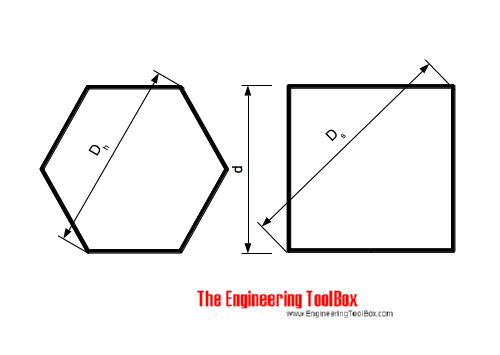Engineering ToolBox - Resources, Tools and Basic Information for Engineering and Design of Technical Applications!

# Hexagons and Squares - Diagonal Lengths

## Distances between corners for hexagons and squares.### Hexagon

The distance between the corners in a hexagon as indicated in the figure can be expressed as

Dh = (2 / 3) 31/2 d

= 1.154701 d                            (1)

### Square

The distance between corners - length of diagonal - in a square can be expressed as

Ds = 21/2 d

= 1.4142 d                            (2)

### Example - Distance between Corners in a Hexagon

The distance between corners in a hexagon where d = 0.4 m can be calculated as

Dh = 1.154701 0.4

= 0.462 m

## Related Topics

• ### Mathematics

Mathematical rules and laws - numbers, areas, volumes, exponents, trigonometric functions and more.

## Related Documents

• ### Centroids of Plane Areas

The controid of square, rectangle, circle, semi-circle and right-angled triangle.
• ### Elementary Curves

Ellipse, circle, hyperbola, parabola, parallel, intersecting and coincident lines.
• ### Equal Areas - Circles vs. Squares

Radius and side lengths of equal areas, circles and squares.
• ### Geometric Shapes - Areas

Areas, diagonals and more - of geometric figures like rectangles, triangles, trapezoids ..
• ### Metric Bolt Heads and Wrench Sizes

ANSI/SO, DIN and JIS bolts.
• ### Regular Polygons

Areas of regular polygons - polygons with 3 to 12 sides.

## Engineering ToolBox - SketchUp Extension - Online 3D modeling!

Add standard and customized parametric components - like flange beams, lumbers, piping, stairs and more - to your Sketchup model with the Engineering ToolBox - SketchUp Extension - enabled for use with older versions of the amazing SketchUp Make and the newer "up to date" SketchUp Pro . Add the Engineering ToolBox extension to your SketchUp Make/Pro from the Extension Warehouse !

We don't collect information from our users. More about

## Citation

• The Engineering ToolBox (2014). Hexagons and Squares - Diagonal Lengths. [online] Available at: https://www.engineeringtoolbox.com/hexagon-square-distance-corner-d_1892.html [Accessed Day Month Year].

Modify the access date according your visit.

9.19.12